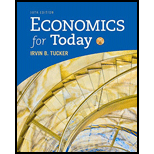# Which of the following is the second step in the model-building process? a. Collect data and test the model. b. Develop a model based on simplified assumptions. c. Identify the problem. d. Include all possible variables that affect the model.### Economics For Today

10th Edition
Tucker
Publisher: Cengage Learning
ISBN: 9781337613040

#### Solutions

Chapter
Section### Economics For Today

10th Edition
Tucker
Publisher: Cengage Learning
ISBN: 9781337613040
Chapter 1, Problem 18SQ
Textbook Problem
5 views

## Which of the following is the second step in the model-building process? a. Collect data and test the model. b. Develop a model based on simplified assumptions. c. Identify the problem. d. Include all possible variables that affect the model.

To determine

The second step in the model building process.

### Explanation of Solution

The models in the economics are small samples of the economy itself. The models helps to form a place where the economists could easily test their economic theories in an actual economy (like atmosphere), which is impossible to study in the open real world.

Option (b):

Identification of the problem in the economy that has to be studied is the first step in the economic model building. To develop a model based on simple assumptions is the step that follows the identification of the problem, and testing the model is the last step in model building. Therefore, it means that it is the second step in the model building. Hence, option 'b' is correct.

Option (a):

The economic modeling is the process of making a small replication of the real economy where the economists experiment the economic theories and collect data from the experiment and analyze it to test the method. The identification of the problem, developing a model based on simple assumptions are done before the collection of data, and testing of the model is the last step in the model building process...

### Still sussing out bartleby?

Check out a sample textbook solution.

See a sample solution

#### The Solution to Your Study Problems

Bartleby provides explanations to thousands of textbook problems written by our experts, many with advanced degrees!

Get Started

Find more solutions based on key concepts
Explain why an economys income must equal its expenditure.

Principles of Macroeconomics (MindTap Course List)

Integrated Case NEW WORLD CHEMICALS INC. 16-16 FINANCIAL FORECASTING Sue Wilson, the new financial manager of N...

Fundamentals of Financial Management, Concise Edition (with Thomson ONE - Business School Edition, 1 term (6 months) Printed Access Card) (MindTap Course List)

A trade discount is a reduction from the list or catalog price offered to different classes of customers.

College Accounting, Chapters 1-27 (New in Accounting from Heintz and Parry)